# 前言

（2020年4月12日更新）过了几年回头看这篇博客，连我自己都看的头发都乱了，又重新理了一遍才把自己说通。。于是这篇博客又被我大改了一遍。

ps. 本问题由淡一抹夕霞提供。

DirectX11 With Windows SDK完整目录

# 一些线性代数基础

## 行主序矩阵与列主序矩阵

$m_{11} \; m_{12} \; m_{13} \; m_{14} \; m_{21} \; m_{22} \; m_{23} \; m_{24} \; m_{31} \; m_{32} \; m_{33} \; m_{34} \; m_{41} \; m_{42} \; m_{43} \; m_{44}$

$\mathbf{M}=\begin{bmatrix} m_{11} & m_{12} & m_{13} & m_{14} \\m_{21} & m_{22} & m_{23} & m_{24} \\m_{31} & m_{32} & m_{33} & m_{34}\\m_{41} & m_{42} & m_{43} & m_{44}\end{bmatrix}$

$\mathbf{M}=\begin{bmatrix} m_{11} & m_{21} & m_{31} & m_{41} \\m_{12} & m_{22} & m_{32} & m_{42} \\m_{13} & m_{23} & m_{33} & m_{43}\\m_{14} & m_{24} & m_{34} & m_{44} \end{bmatrix}$

## 矩阵左乘与右乘

ps. 向量也是矩阵

$\mathbf{(vM)}^{T} = \mathbf{M}^{T} \mathbf{v}^{T}$

# C++和HLSL中矩阵的内存布局

$m_{11} \; m_{12} \; m_{13} \; m_{14} \; m_{21} \; m_{22} \; m_{23} \; m_{24} \; m_{31} \; m_{32} \; m_{33} \; m_{34} \; m_{41} \; m_{42} \; m_{43} \; m_{44}$

cb0.xyzw = (m11, m12, m13, m14);
cb0.xyzw = (m21, m22, m23, m24);
cb0.xyzw = (m31, m32, m33, m34);
cb0.xyzw = (m41, m42, m43, m44);


cbuffer cb : register(b0)
{
(row_major) matrix g_World;
}


$\begin{bmatrix} m_{11} & m_{21} & m_{31} & m_{41} \\ m_{12} & m_{22} & m_{32} & m_{42} \\ m_{13} & m_{23} & m_{33} & m_{43} \\ m_{14} & m_{24} & m_{34} & m_{44} \\ \end{bmatrix}$

$\begin{bmatrix}m_{11} & m_{12} & m_{13} & m_{14} \\m_{21} & m_{22} & m_{23} & m_{24} \\m_{31} & m_{32} & m_{33} & m_{34} \\m_{41} & m_{42} & m_{43} & m_{44} \\\end{bmatrix}$

# HLSL中的mul函数

## dp4指令

dp4是一个汇编指令(微软官方文档链接)，使用方法如下：

dp4 dst, src0, src1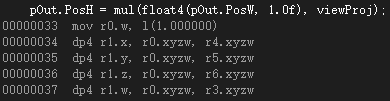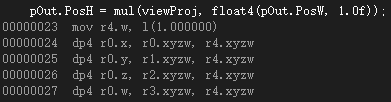cbuffer cb : register(b0)
{
row_major matrix gWorld;
row_major matrix gView;
row_major matrix gProj;
}

struct VertexPosNormalTex
{
float3 PosL : POSITION;
float3 NormalL : NORMAL;
float2 Tex : TEXCOORD;
};

struct VertexPosHWNormalTex
{
float4 PosH : SV_POSITION;
float3 PosW : POSITION; // 在世界中的位置
float3 NormalW : NORMAL; // 法向量在世界中的方向
float2 Tex : TEXCOORD;
};

// 顶点着色器
VertexPosHWNormalTex VS(VertexPosNormalTex pIn)
{
VertexPosHWNormalTex pOut;

row_major matrix viewProj = mul(gView, gProj);

pOut.PosW = mul(float4(pIn.PosL, 1.0f), gWorld).xyz;
pOut.PosH = mul(float4(pOut.PosW, 1.0f), viewProj);
pOut.NormalW = mul(pIn.NormalL, (float3x3) gWorldInvTranspose);
pOut.Tex = pIn.Tex;
return pOut;
}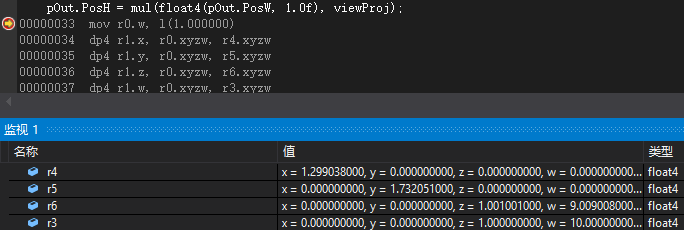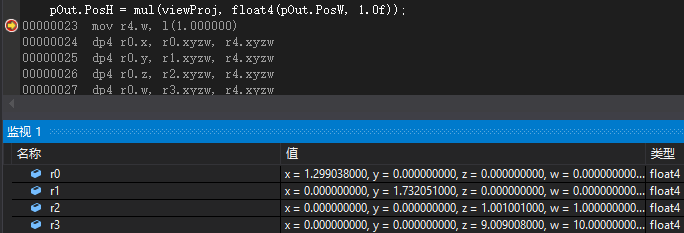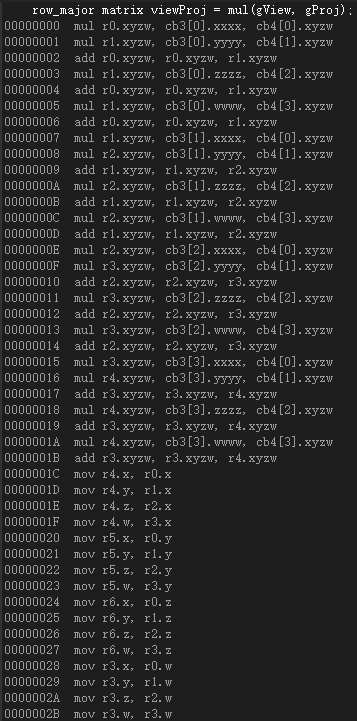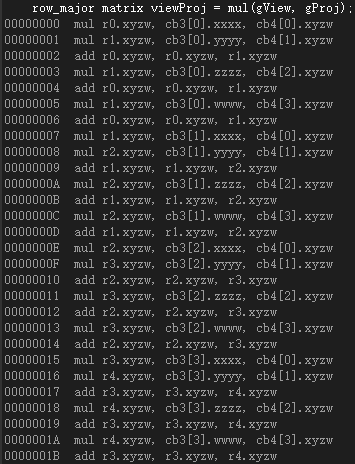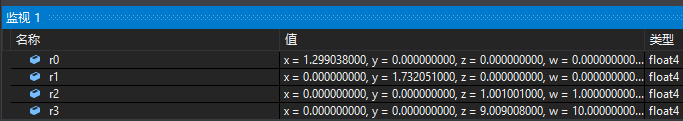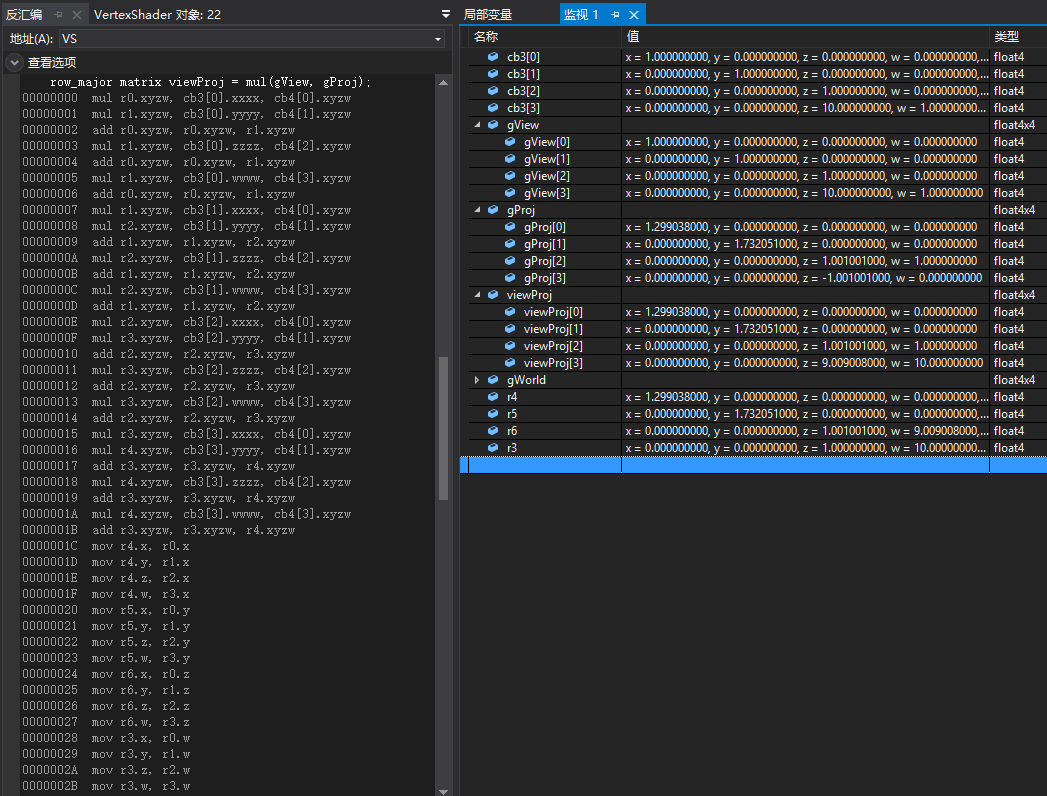# 总结

1. C++代码端的转置
2. HLSL中matrix(float4x4)是列主矩阵时会发生转置
3. mul乘法内部是以列向量右乘矩阵的形式实现的，对于行向量左乘矩阵的情况会发生转置

1. C++代码端不进行转置，HLSL中使用row_major matrix(行主序矩阵)，mul函数让向量放在左边(行向量)，这样实际运算就是(行向量 X 行主序矩阵) 。这种方法易于理解，但是这样做dp4运算取矩阵的列很不方便，在HLSL中会产生用于转置矩阵的大量指令，性能上有损失。
2. C++代码端进行转置，HLSL中使用matrix(列主序矩阵) ，mul函数让向量放在左边(行向量)，这样就是(行向量 X 列主序矩阵)，但C++这边需要进行一次矩阵转置，HLSL内部不产生转置 。这是官方例程所使用的方式，这样可以使得dp4运算可以直接取列主序矩阵的行，从而避免内部产生大量的转置指令。后续我会将教程的项目也使用这种方式。
3. C++代码端不进行转置，HLSL中使用matrix(列主序矩阵)，mul函数让向量放在右边(列向量)，实际运算是(列主序矩阵 X 列向量)。这种方法的确可行，取列矩阵的行也比较方便，效率上又和2等同，就是HLSL那边的矩阵乘法都要反过来写，然而DX本身就是崇尚行主矩阵的，把OpenGL的习惯带来这边有点。。。
4. C++代码端进行转置，HLSL中使用row_major matrix(行主序矩阵)，mul函数让向量放在右边(列向量)，实际运算是(行主序矩阵 X 列向量)。 就算这种方法也可以绘制出来，但还是很让人难受，比第2点还难受，我甚至不想去说它。

DirectX11 With Windows SDK完整目录

posted @ 2018-10-19 02:57  X_Jun  阅读(4457)  评论(4编辑  收藏  举报
levels of contents